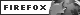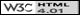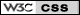# Principles of Linear Algebra with Mathematica®## Info and Errata

### Contact Info

If you have any comments, suggestions, critiques, or have found errors of any kind in this text, we would love to hear from you. We are always looking for new ideas which combines topics from linear algebra and Mathematica. Note that we have an "Extra Topics" portion of this page for just a couple of the ideas that we could not fit in the text due to page constraints. To contact us, simply click on "Contact us", which is located at the lower left of any page on this site (including this one).

### Errata

As much as we would like the text to be error free, undoubtedly it is not. Below is a link to an errata document which will help clarify any issues we have found (or have been told about) in this text.

Linear algebra is a relatively new area of mathematics, whose concepts were formulated starting in the late nineteenth century. A few of the common topics one normally encounters in linear algebra are vectors, matrices and linear maps. These areas of study have wide ranging applications in physics, the natural and social sciences, and serve as a stepping stone for studying nonlinear systems that are constructed to model real world phenomena.

Principles of Linear Algebra with Mathematica is an attempt to cross the gap between beginning linear algebra and the computational and geometrical linear algebra one encounters more frequently in applied settings. Many topics in linear algebra are quite simple, but can be computationally intensive. Computer algebra systems, such as Mathematica, are important in that they can be used to apply simple concepts to computationally challenging problems. Furthermore, the graphical abilities of Mathematica help to visualize many of the geometric aspects of linear algebra. The book itself is a self-contained first course in the subject areas already mentioned as well as many others. Additionally, topics such as: sensitivity of solutions to errors in systems of equations and rotations of geometric objects in the plane and in space, can only be covered with the use of a computer algebra system.

An attempt is also made to use the full power of Mathematica: graphics in two and three dimensions, animations, symbolic manipulations, numerical computations and programming, to name just a few. In the text, we include all of the Mathematica commands required to solve complex and computationally challenging linear algebra problems.

This is not just a theory-proof-problem text; we explore topics in a very reader friendly and natural way. Our approach to presenting the material has made the text very readable, and has made the use of Mathematica to solve problems fun and less tedious. All of these facts make this book current, useful and interesting beyond the standard scope of a linear algebra text, while still giving the reader an understanding of the underlying fundamental concepts that make up the field of linear algebra.

We hope that students and educators alike will take the time to utilize Mathematica in their explorations of the various topics covered in this text.

Contact us | © K. Frinkle and K. Shiskowski | best viewed with||